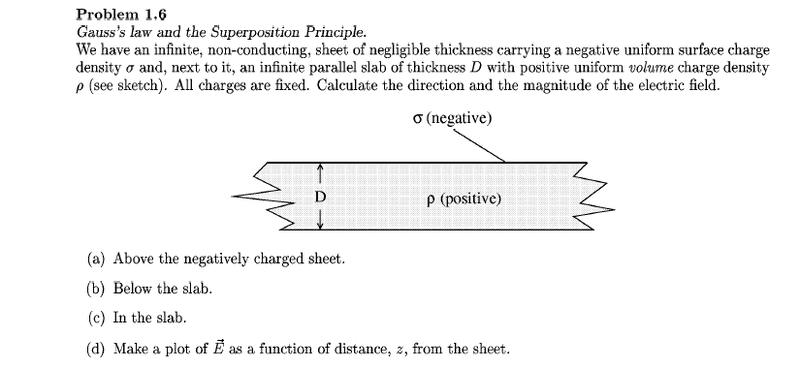# Confusion to the solution of a MIT ocw problem (1 Viewer)

### Users Who Are Viewing This Thread (Users: 0, Guests: 1)

#### JYangQi00

1. The problem statement, all variables and given/known data
My snipping tool is totally broken. So i'm going to leave a link :x
It's problem 1.6
http://ocw.mit.edu/courses/physics/8-02-electricity-and-magnetism-spring-2002/assignments/ps1a.pdf

2. Relevant equations

$E_{sheet}$=$\frac{σ}{2ε_{0}}$
$E_{slab}$=$\frac{ρD}{2ε_{0}}$
$\frac{Q}{ε_{0}}$=$\oint$EdA

3. The attempt at a solution

I got everything on the solutions page (http://ocw.mit.edu/courses/physics/8-02-electricity-and-magnetism-spring-2002/assignments/pss1.pdf) But I can't seem to understand why region I of Esheet is positive and Regions II and III are negative. If σ is a negative charge, shouldn't the signs be switched around?

#### majormaaz

It's interesting that you have Gauss's Law as one of your equations. When taking a look at the plane, have you thought about it from a flux perspective?

#### SammyS

Staff Emeritus
Homework Helper
Gold Member
1. The problem statement, all variables and given/known data
My snipping tool is totally broken. So i'm going to leave a link :x
It's problem 1.6
http://ocw.mit.edu/courses/physics/8-02-electricity-and-magnetism-spring-2002/assignments/ps1a.pdf

2. Relevant equations

$E_{sheet}$=$\frac{σ}{2ε_{0}}$
$E_{slab}$=$\frac{ρD}{2ε_{0}}$
$\frac{Q}{ε_{0}}$=$\oint$EdA

3. The attempt at a solution

I got everything on the solutions page (http://ocw.mit.edu/courses/physics/8-02-electricity-and-magnetism-spring-2002/assignments/pss1.pdf) But I can't seem to understand why region I of Esheet is positive and Regions II and III are negative. If σ is a negative charge, shouldn't the signs be switched around?
Welcome to PF !

Here is an image of the problem:#### Attachments

• 40.6 KB Views: 169

#### rude man

Homework Helper
Gold Member
Post #2 is a good hint, follow it!
3 equations, 3 unknowns, 3 gaussian surfaces.
Hint: make sure two of them line up correctly!

#### JYangQi00

Welcome to PF !

Here is an image of the problem:Thanks! My snipping tool makes it so that the screen turns gray and I snip black images >_<

### The Physics Forums Way

We Value Quality
• Topics based on mainstream science
• Proper English grammar and spelling
We Value Civility
• Positive and compassionate attitudes
• Patience while debating
We Value Productivity
• Disciplined to remain on-topic
• Recognition of own weaknesses
• Solo and co-op problem solving